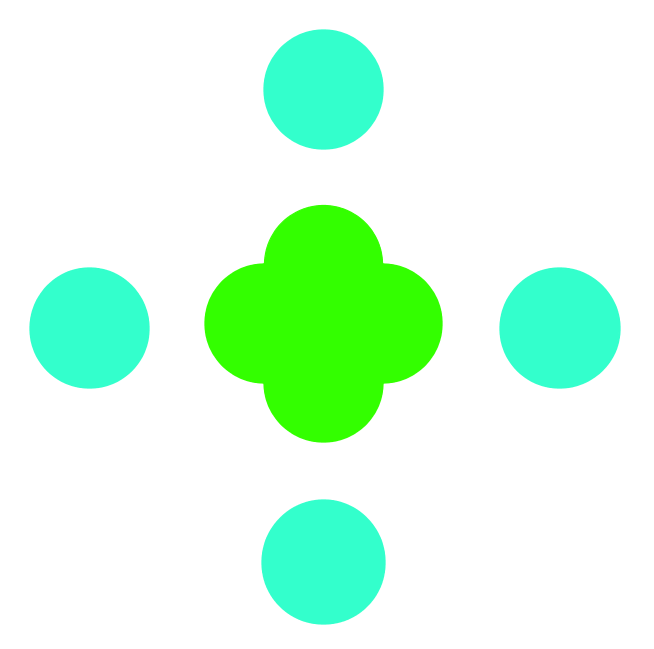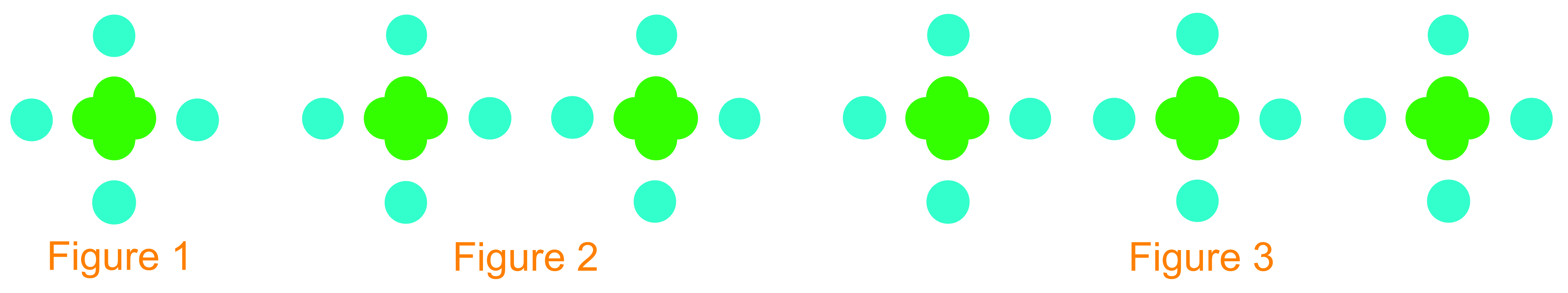# Patterns

### Patterns

In this section, we are asked to describe given patterns and copy and complete tables of values for these patterns. Also, we use variables to write expressions for the patterns and then solve for these variables. In this section, we will gain fundamental knowledge about expressions and variables that we will build on in other sections.

#### Lessons

• 1.
A bedroom wall is painted with blue polka dots and green flowers. The basic design is shown.Below are two patterns made when 2 and 3 of the basic designs are put together.a)
Create a table that shows the pattern of blue polka dots and green flowers for the first 6 figures. Describe the pattern.

b)
Use b to represent the number of green flowers. Write an expression to describe the number of blue polka dots.

c)
How many polka dots will there be if there are 32 flowers?

• 2.
Bianca was changing fractions into decimal numbers and noticed a pattern.
$\frac{1}{11}=0.0909...$
$\frac{2}{11}=0.1818...$
$\frac{3}{11}=0.2727...$
a)
Describe the pattern.

b)
Write $\frac{4}{11}$ as a decimal number, using bar notation.

c)
Write $0.54545454...$ as a fraction.

• 3.
Bella always sleeps for 8 hours on school days and 2 extra hours on holidays.
a)
Write an expression to show the hours of sleep that Bella gets in a week.

b)
If Bella only has 2 days of school this week, how many hours of sleep does she have for the whole week? Use the expression in the previous part to answer this question.